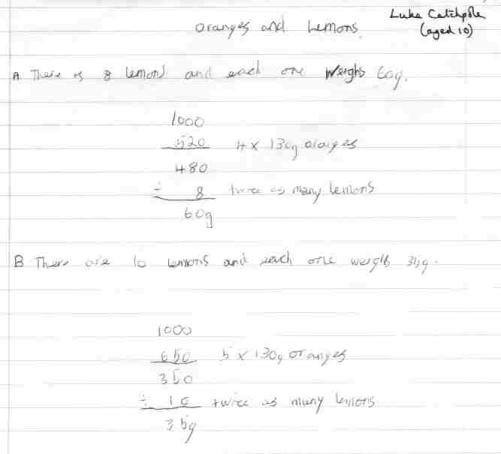#### You may also like### Exploring Wild & Wonderful Number Patterns

EWWNP means Exploring Wild and Wonderful Number Patterns Created by Yourself! Investigate what happens if we create number patterns using some simple rules.### Sending Cards

This challenge asks you to investigate the total number of cards that would be sent if four children send one to all three others. How many would be sent if there were five children? Six?### Dice and Spinner Numbers

If you had any number of ordinary dice, what are the possible ways of making their totals 6? What would the product of the dice be each time?

# Oranges and Lemons

##### Age 7 to 11Challenge Level

Steffi, Emma and Amelia from Girton Glebe Primary School near Cambridge. We think we have the answer:

We think that the answer is $8$ lemons, which have a mass of $60$g, and $4$ oranges.

What strategy did the girls use to arrive at that answer? They explain:

We got the answer by trial and improvement.

That last point is very important! All the people who wrote in agree with the girls. But Luke shows his calculations to prove to us that there could be more than one possible answer. Do you agree with Luke?Pupils at Alice Smith International School in Kuala Lumpur, Malaysia also worked on the Oranges and Lemons problem. They agree with Luke. One of the pupils, Kevin, shows all of the possibilities. They prove that there are in fact two possibilities but that there are no more.

Weights = $1$ orange weighs $130$g. $1$ lemon weighs less than $87$g.

To make it easy to read the answers, the pupils use a table:

Number of oranges Number of lemons Right / Wrong
$1$ $2$ Wrong
$2$ $4$ Wrong
$3$ $6$ Wrong
$4$ $8$ Right
$5$ $10$ Right
$6$ $12$ Wrong
$7$ $14$ Wrong
$8$ $16$ Wrong

To show how they arrived at each of these answers, here are the calculations:

1. $130$g + ($87$g $\times$ $2$) $87$g is max weight for Lemons = $304$g
2. ($130$g $\times$ $2$) + ($87$g $\times$ $4$) = $608$g
3. ($130$g $\times$ $3$) + ($87$g $\times$ $6$) = $912$g
4. ($130$g $\times$ $4$) + ($60$g $\times$ $8$) = $1000$g or $1$kg
5. ($130$g $\times$ $5$) + ($35$g $\times$ $10$) = $1000$g or $1$kg
6. ($130$g $\times$ $6$) = $780$g. $1000$g - $780$g = $220$g. $220$g divided by $12$ is $18.3$ recurring. It isn't a whole number.
7. $130$g $\times$ $7$ = $910$g. $1000$g - $910$g = $90$g. $90$ divided by $14$ equals $6.428571429$. It isn't a whole number.
8. $130$g $\times$ $8$ = $1040$g. It is more than $1000$g or $1$kg